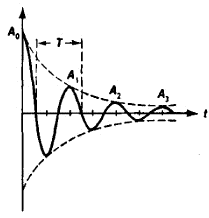# Damping of Oscillations

The following article is from The Great Soviet Encyclopedia (1979). It might be outdated or ideologically biased.

## Damping of Oscillations

a decrease in intensity of oscillations with time caused by energy losses in the oscilla-tory system. The simplest case of energy loss for an oscillation is the conversion of energy into heat as a result of friction in mechanical systems and of resistance in electrical systems. In electrical systems, damping of oscillations may also be due to the radiation of electromagnetic energy.

The law of damping of oscillations is determined by the nature of the energy loss and other properties of the system. The case that has been studied most thoroughly is the damping of oscillations caused by reduction in energy that is proportional to the square of the motion velocity in a mechanical system or, correspondingly, to the square of the current value in an electrical system; it is valid for linear systems. The law is then exponential—that is, the peak-to-peak values of the oscillations decrease according to the law of a geometric progression (see Figure 1).Figure 1. Decay of oscillations: (A0) initial amplitude, (T) period

The energy losses in a system that cause damping of oscillations disturb their periodicity, so that the damped oscillations are not a periodic process and, strictly speaking, the concepts of period and frequency are not applicable to them. However, when damping is small, the conditions of the system repeat approximately, so that it is possible arbitrarily to use the concept of a period as the time interval between two successive passages of an oscillating physical quantity (current, voltage, peak-to-peak vibrations of a pendulum, and so on) in the same direction through the maximum value. An evaluation of the relative decrease in the amplitude of the oscillations during a period gives the logarithmic decrement. The damping rate is related to the quality of the oscillatory system.